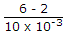Electronics and Communication Engineering - Electronic Devices and Circuits

16.

An LED has a rating of 2 V and 10 mA. It is used along with 6V battery. The range of series resistance is

 A. 0 to 200 Ω B. 200 - 400 Ω C. 200 Ω and above D. 400 Ω and above

Explanation:

R == 400Ω.

R must be at least 400Ω so that current in LED does not exceed 10 mA.

17.

The number of doped regions in PIN diode is

 A. 1 B. 2 C. 3 D. 1 or 2

Explanation:

A PIN diode has p and n doped regions separated by intrinsic layer.

18.

A transistor has two p-n junctions. The batteries should be connected such that

 A. both junctions are forward biased B. both junctions are reverse biased C. one junction is forward biased and the other is reverse biased D. none of the above

Explanation:

Emitter-base junction is forward biased and base collector junction is reverse biased.

19.

A silicon (PN) junction at a temperature of 20°C has a reverse saturation current of 10 pico Ampere. The reverse saturation current at 40°C for the same bias is approximately.

 A. 30 pA B. 40 pA C. 50 pA D. 60 pA

Explanation:

By increasing of temperature by 10°C, Io become double so by increasing temperature 20°C, Io become 4 time than initial value... and it is 40 PA.

20.

In a bipolar transistor the barrier potential

 A. 0 B. a total of 0.7 V C. 0.7 V across each depletion layer D. 0.35 V